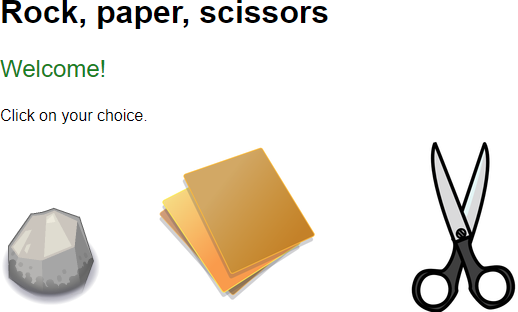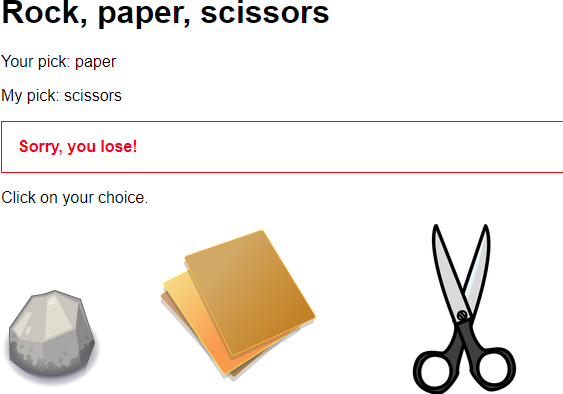# Fun: RPS cheater

Let's make a rock-paper-scissors game, where the computer always wins. You can try it

The program uses the GET parameter `pick` to get the user's choice. If `pick` isn't there...

`rock-paper-scissors.php`

... the program prints a welcome message:If there's a user pick...

`rock-paper-scissors.php?pick=paper`

... the program shows the user's pick, and one of its own. Thing is, it always wins.### Making a choice

Here's the first part of the program:

1. <?php
2. \$userPick = null;
3. \$computerPick = null;
4. if (isset(\$_GET['pick'])) {
5.     \$userPick = \$_GET['pick'];
6.     if (\$userPick == 'rock') {
7.         \$computerPick = 'paper';
8.     }
9.     if (\$userPick == 'paper') {
10.         \$computerPick = 'scissors';
11.     }
12.     if (\$userPick == 'scissors') {
13.         \$computerPick = 'rock';
14.     }
15. }
16. ?>

The variables `\$userPick` and `\$computerPick` record the user's and computer's pick. They start off as null. That's how the program will know whether it needs to output the welcome text.

If there is a value for `pick` (line 4), the code grabs it, and puts it into `\$userPick` (line 5). Then it makes its own choice. It will take whatever beats the user.

### Showing the results

The rest of the program tells the user what happened.

1. <h1>Rock, paper, scissors</h1>
2. <?php
3. if (\$userPick == null) {
4.     print "<p class='welcome'>Welcome!</p>";
5. }
6. else
7. {
9.     print "<p>My pick: \$computerPick</p>\n";
10.     print "<p class='lose'>Sorry, you lose!</p>\n";
11. }
12. ?>
14. <p>
15.     <a class="pick" href="rock-paper-scissors.php?pick=rock">
16.         <img src="rock.png" alt="Rock">
17.     </a>
18.     <a class="pick" href="rock-paper-scissors.php?pick=paper">
19.         <img src="papers.png" alt="Paper">
20.     </a>
21.     <a class="pick" href="rock-paper-scissors.php?pick=scissors">
22.         <img src="scissors.png" alt="Scissors">
23.     </a>
24. </p>

First, the code decides whether to show the welcome message. It will do that if `\$userPick` is MT.

• if (\$userPick == null) {
•     print "<p class='welcome'>Welcome!</p>";
• }
• else
• {

If `\$userPick` isn't null, then the program shows the two picks, and who won:

• print "<p>My pick: \$computerPick</p>\n";
• print "<p class='lose'>Sorry, you lose!</p>\n";

There's no need for an `if` to detect who won. The computer always wins.

Finally, the program shows the three choices, for the next game:

• <p>
•     <a class="pick" href="rock-paper-scissors.php?pick=rock">
•         <img src="rock.png" alt="Rock">
•     </a>
•     <a class="pick" href="rock-paper-scissors.php?pick=paper">
•         <img src="papers.png" alt="Paper">
•     </a>
•     <a class="pick" href="rock-paper-scissors.php?pick=scissors">
•         <img src="scissors.png" alt="Scissors">
•     </a>
• </p>

Notice the `href`s for the links. The value of the pick that matches the image is at the end of the URL.

### Easy peasy

That's it! See how easy it is for a computer to cheat? We could make it less obvious, by cheating on, say, one game in four.

Yeah, online gambling's legit, right? What could possibly go wrong?